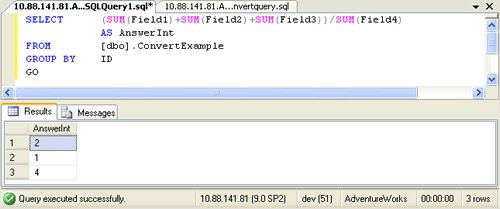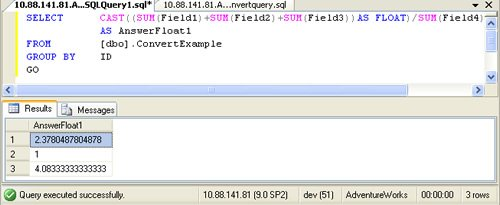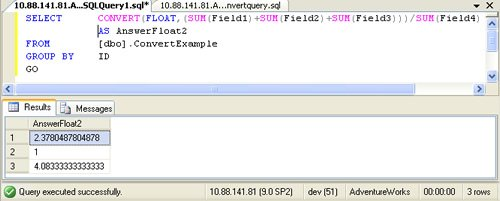# SQL SERVER – Get Answer in Float When Dividing of Two Integer

Many times we have requirements of some calculations amongst different fields in Tables. One of the software developers here was trying to calculate some fields having integer values and divide it which gave incorrect results in integer where accurate results including decimals was expected.

Something as follows,

Example,

```USE [AdventureWorks]
GO
CREATE TABLE [dbo].ConvertExample(
[ID]        [int] NULL,
[Field1]    [int] NULL,
[Field2]    [int] NULL,
[Field3]    [int] NULL,
[Field4]    [int] NULL
)
GO
INSERT INTO [dbo].ConvertExample
VALUES (1,30,40,60,80)
GO
INSERT INTO [dbo].ConvertExample
VALUES (2,20,10,50,80)
GO
INSERT INTO [dbo].ConvertExample
VALUES (3,15,140,90,60)
GO
INSERT INTO [dbo].ConvertExample
VALUES (1,60,0,5,2)
GO
SELECT *
FROM [dbo].ConvertExample
GO
SELECT (SUM(Field1)+SUM(Field2)+SUM(Field3))/SUM(Field4)
FROM [dbo].ConvertExample
GROUP BY ID
GO
```However, We need to CAST or CONVERT the numerator to solve this or we can say that to get the results we wanted.

Example using CAST

```SELECT CAST((SUM(Field1)+SUM(Field2)+SUM(Field3))AS FLOAT)/SUM(Field4) AS AnswerFloat1
FROM [dbo].ConvertExample
GROUP BY ID
GO
```Example Using CONVERT:

```SELECT CONVERT(FLOAT,(SUM(Field1)+SUM(Field2)+SUM(Field3)))/SUM(Field4) AS AnswerFloat2
FROM [dbo].ConvertExample
GROUP BY ID
GO
```Conclusion

When we expect the results in floating or decimals then we need to convert or cast the numerator part to get the accurate results with FLOAT or DECIMAL Whichever needed.

Reference : Pinal Dave (https://blog.sqlauthority.com)

#### Related Posts

•Jacob sebastian
September 26, 2008 2:40 pm

Nice post and something that is very much useful in the day-to-day programming life.

Just wanted to add to what is already explained, that, SQL Server automatically does a cast to the data type having the highest precedence. So the result of INT and INT will be INT, but INT and FLOAT will be FLOAT because FLOAT has a higher precedence. If you want a different data type, you need to do an EXPLICIT cast.

For example:

SELECT 100/3 AS Result
/*
Result
———–
33
*/

SELECT 100/3.0 AS Result
/*
Result
—————————————
33.333333
*/

The precedence of each data type is documented here: https://docs.microsoft.com/en-us/previous-versions/sql/sql-server-2005/ms190309(v=sql.90)

• Thank you Jacob,

I like your additional explanation. I just realize after reading your explanation that I should include your explanation in the post itself so it is visible to everybody right away.

Also this has brought another idea in my mind that when creating calculated field the same scenario happens. E.g. When we create calculated field from additions of INT it will give us final calculated field as INT. If we want that in another datatype we should have either follow your suggestion or CAST them.

Regards,
Pinal

•Jerry Hung
September 26, 2008 7:53 pm

Sometimes I get lazy and just do
field = int column A / int column B * 1.0

Then I *think* I get float back

•Jorgen Harmse
March 14, 2013 10:11 pm

Certainly this produces a float, but the value depends on precedence of / & *, which is non-obvious to casual readers. I would specify A / (B * 1.0) or (A / B) * 1.0 or A * 1.0 / B. The first & third do what the original questioner wanted. The second does integer division and turns the answer into a float.

•Jorgen Harmse
March 14, 2013 10:28 pm

Certainly it produces a float, but I don’t think the value is what the original post wanted. The integer divide has to happen first, and the result of that is converted to float. A / (B * 1.0) and A * 1.0 / B b both perform floating point division.

> sqldf(“select 8 / 3 * 1.0”)
8 / 3 * 1.0
1 2

•Marek Śliwiński
September 28, 2008 3:09 pm

Thanks guys, you make a nice duet together :)

•Darren
July 21, 2009 7:53 pm

This has caught me many times…
Putting the 1.0 at the beginning of the line makes a difference:

declare @a int,@b int,@c float
set @a=1
set @b=2

select @a/@b – Result 0
select @a/@b*1.0 – Result 0.0
select 1.0*@a/@b – Result 0.500000

•Happy Web Designer
December 11, 2009 1:08 pm

Excellent,

To get a percentage with decimal places

100.0*@numerator/@denominator

where both are INT and extract as float.

Job done.

Thank you.

•Nowfal majeed
March 7, 2012 4:29 pm

Thank You very much for this examples..I have tried i got answer..But one thing if i need 1.20 instead of 1.2089876 what i have to do?

•March 20, 2012 5:44 pm

select round(col,2)

•Jesse Anderson
October 15, 2012 8:10 pm

Thanks for the post Dave et al. I must say, after beginning my professional career as a developer, your blog has been a top reference of mine (along with MSDN of ciurse) when I need help solving a problem with SQL.

•Mahesh Chikhale
February 2, 2013 5:04 pm

hello sir my table is able to store the float value like 75.5 but if i am going to store like 125.00,50.00 etc it will store 125 , 50 not giving me decimal places
what is the solution if i want to store the values with 00 in decimal places?

•avi
April 29, 2013 4:17 pm

thank you better than msdn

•JM
November 26, 2013 2:35 pm

Thank you so much for your post, i’m learning on MSSQL and have been searching how to sum multiple columns, divide it and get a result with required decimal point…your blog has again answered to my questions and I always got answers from your blog…good blog and best blog so far IMHO…

A BIG THANK AGAIN.

JM

•ashwani bhardwaj
April 15, 2015 6:14 pm

thanks so much.. because of your example my tool resolution has been done

•Nikolay Sonin
September 13, 2019 3:17 am

Hi,

Thanks for the post, but the cast does not seem to work as it always should. Here’s my example of its weird behavior:

create function [dbo].[F_1]()
returns @result table
(
RateVacancy decimal
)
as
begin

insert into @result
select
convert(decimal, 20) / convert(decimal, 30 ) as RateVacancy
return
end
go

Now this query

select * from dbo.F_1()

returns 1 instead of expected 0.66666666. I wonder why cast to decimal doesn’t work in this case at all.

•mnarayan777
December 18, 2019 3:38 am

I have a case where there are certain rows like A, B ,C. Now i have to see if there is B in the table then divide all the cols corresponding to B with C and display it in a seprate column . Is there a simple way to do that

•